Recently, a fellow in category land discovered a fact that we in Haskell land have actually known for a while (in addition to things most of us probably don't). Specifically, given two categories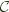and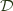, a functor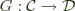, and provided some conditions inhold, there exists a monad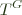, the codensity monad of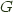.

In category theory, the codensity monad is given by the rather frightening expression: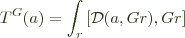Where the integral notation denotes an end, and the square brackets denote a power, which allows us to take what is essentially an exponential of the objects ofby objects of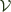, whereis enriched in. Provided the above end exists,is a monad regardless of whetherhas an adjoint, which is the usual way one thinks of functors (in general) giving rise to monads.

It also turns out that this construction is a sort of generalization of the adjunction case. If we do have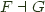, this gives rise to a monad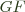. But, in such a case,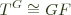, so the codensity monad is the same as the monad given by the adjunction when it exists, but codensity may exist when there is no adjunction.

In Haskell, this all becomes a bit simpler (to my eyes, at least). Our categoryis always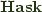, which is enriched in itself, so powers are just function spaces. And all the functors we write will be rather like(objects will come from kinds we can quantify over), so ends of functors will look like forall r. F r r where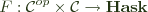. Then:
 newtype Codensity f a = Codensity (forall r. (a -> f r) -> f r) 

As mentioned, we've known for a while that we can write a Monad instance for Codensity f without caring at all about f.

As for the adjunction correspondence, consider the adjunction between products and exponentials: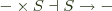This gives rise to the monad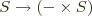, the state monad. According to the facts above, we should have that Codensity (s ->) (excuse the sectioning) is the same as state, and if we look, we see:
 forall r. (a -> s -> r) -> s -> r 

which is the continuation passing, or Church (or Boehm-Berarducci) encoding of the monad.

Now, it's also well known that for any monad, we can construct an adjunction that gives rise to it. There are multiple ways to do this, but the most accessible in Haskell is probably via the Kleisli category. So, given a monad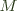on, there is a category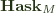with the same objects, but where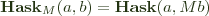. The identity for each object is return and composition of arrows is:
 (f >=> g) x = f x >>= g 

Our two functors are:
 F a = a F f = return . f

 

U a = M a U f = (>>= f) 

Verifying that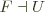requires only that, but this is just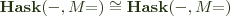, which is a triviality. Now we should have that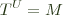.

So, one of the simplest monads is reader,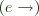. Now,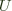just takes objects in the Kleisli category (which are objects in) and appliesto them, so we should have Codensity (e ->) is reader. But earlier we had Codensity (e ->) was state. So reader is state, right?

We can actually arrive at this result another way. One of the most famous pieces of category theory is the Yoneda lemma, which states that the following correspondence holds for any functor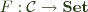: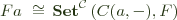This also works for any functor intoand looks like:
 F a ~= forall r. (a -> r) -> F r 

for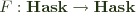. But we also have our functor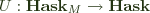, which should look more like:

 U a ~= forall r. (a -> M r) -> U r M a ~= forall r. (a -> M r) -> M r

So, we fill in M = (e ->) and get that reader is isomorphic to state, right? What's going on?

To see, we have to take a closer look at natural transformations. Given two categoriesand, and functors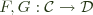, a natural transformation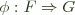is a family of maps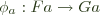such that for every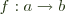the following diagram commutes:The key piece is what the morphisms look like. It's well known that parametricity ensures the naturality of t :: forall a. F a -> G a for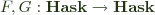, and it also works when the source is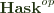. It should also work for a category, call it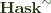, which has Haskell types as objects, but where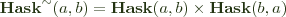, which is the sort of category that newtype Endo a = Endo (a -> a) is a functor from. So we should be at liberty to say:
 Codensity Endo a = forall r. (a -> r -> r) -> r -> r ~= [a] 

However, hom types forare not merely made up ofhom types on the same arguments, so naturality turns out not to be guaranteed. A functor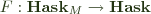must take a Kleisli arrow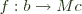to an arrow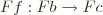, and transformations must commute with that mapping. So, if we look at our use of Yoneda, we are considering transformations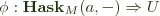:Now,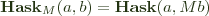and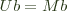. So

t :: forall r. (a -> M r) -> M r

will get us the right type of maps. But, the above commutative square corresponds to the condition that for all f :: b -> M c:
 t . (>=> f) = (>>= f) . t 

So, if we have h :: a -> M b, Kleisli composing it with f and then feeding to t is the same as feeding h to t and then binding the result with f.

Now, if we go back to reader, we can consider the reader morphism:
 f = const id :: a -> e -> e 

For all relevant m and g,  m >>= f = id and g >=> f = f. So the
naturality condition here states that t f = id.

Now, t :: forall r. (a -> e -> r) -> e -> r. The general form of these is state actions (I've split e -> (a, e) into two pieces):

t f e = f (v e) (st e)
where
rd :: e -> a
st :: e -> e


If f = const id, then:

t (const id) e = st e
where
st :: e -> e


But our naturality condition states that this must be the identity, so we must have st = id. That is, the naturality condition selects t for which the corresponding state action does not change the state, meaning it is equivalent to a reader action! Presumably the definition of an end (which involves dinaturality) enforces a similar condition, although I won't work through it, as it'd be rather more complicated.

However, we have learned a lesson. Quantifiers do not necessarily enforce (di)naturality for every category with objects of the relevant kind. It is important to look at the hom types, not just the objects .In this case, the point of failure seems to be the common, extra s. Even though the type contains nautral transformations for the similar functors over, they can (in general) still manipulate the shared parameter in ways that are not natural for the domain in question.

I am unsure of how exactly one could enforce the above condition in (Haskell's) types. For instance, if we consider:

forall r m. Monad m => (a -> m r) -> m r

This still contains transformations of the form:

 t k = k a >> k a

And for this to be natural would require:

 (k >=> f) a >> (k >=> f) a = (k a >> k a) >>= f

Which is not true for all possible instantiations of f. It seems as though leaving m unconstrained would be sufficient, as all that could happen is t feeding a value to k and yielding the result, but it seems likely to be over-restrictive.